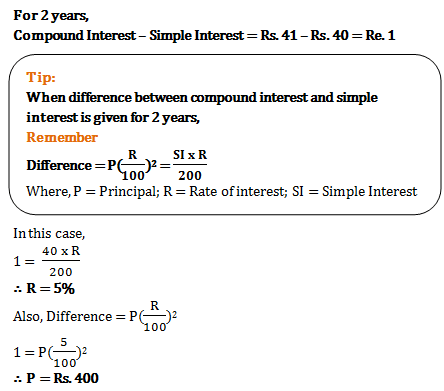# Compound Interest - Quantitative Aptitude (MCQ) questions for Q. 29409

Q.  If at same rate of interest, in 2 years, the simple interest is Rs. 40 and compound interest is Rs. 41, then what is the principal?
- Published on 12 Apr 17

a. Rs. 500
b. Rs. 400
c. Rs. 800
d. Rs. 820